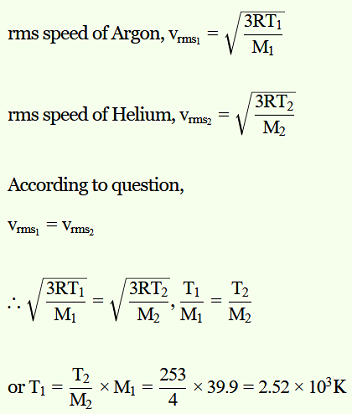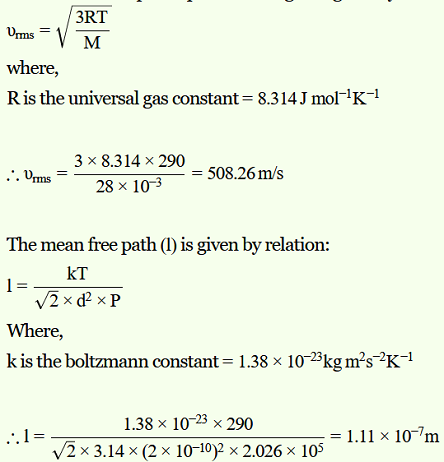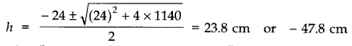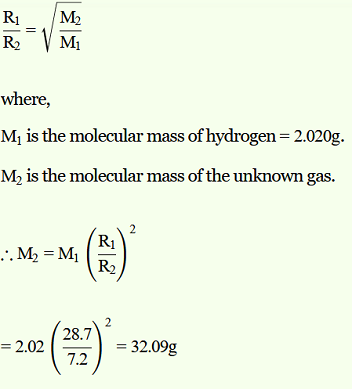# NCERT SOLUTIONS FOR CLASS 11 PHYSICS CHAPTER-13 KINETIC THEORY OF GASES

The NCERT Solution for Class 11  physics includes answers to every question from the NCERT textbook’s exercise. Top students love SWC NCERT Solutions because they are very effective. In general, Class 11 is regarded as the most significant year in a student’s professional development. The NCERT answers for class 11  physics were created with the goal of providing students with the most help possible.

Answers to the class 11  physics questions provided in the exercise might be challenging for students for a number of reasons. One should not omit any NCERT textbook content in order to get the highest possible grade. Use the Swastik Classes’ NCERT answers for physics class 11 as a resource. Important exam-based questions are covered in depth in each chapter.

## NCERT SOLUTIONS FOR CLASS 11 PHYSICS CHAPTER-13 KINETIC THEORY OF GASES – Exercises

### Chapter-13 Kinetic Theory

Question 1. Estimate the fraction of molecular volume to the actual volume occupied by oxygen gas at STP. Take the diameter of an oxygen molecule to be 3Å.

Solution :
Diameter of an oxygen molecule, d = 3Å
Radius, r = d/2 = 3/2 = 1.5 Å = 1.5 × 10–8 cm
Actual volume occupied by 1 mole of oxygen gas at STP = 22400 cm3
Molecular volume of oxygen gas, V = (4/3)πr3N
Where, N is Avogadro’s number = 6.023 × 1023 molecules/mole
∴ V = (4/3) × 3.14 × (1.5 × 10-8)3 × 6.023 × 1023 = 8.51 cm3
Ratio of the molecular volume to the actual volume of oxygen = 8.51 / 22400
= 3.8 × 10-4.

Question 2. Molar volume is the volume occupied by 1 mol of any (ideal) gas at standard temperature and pressure (STP: 1 atmospheric pressure, 0 °C). Show that it is 22.4 litres.

Solution :
The ideal gas equation relating pressure (P), volume (V), and absolute temperature (T) is given as:
PV = nRT
Where,
R is the universal gas constant = 8.314 J mol–1 K–1
n = Number of moles = 1
T = Standard temperature = 273 K
P = Standard pressure = 1 atm = 1.013 × 105 Nm–2
∴ V = nRT / P
= 1 × 8.314 × 273 / (1.013 × 105)
= 0.0224 m3
= 22.4 litres
Hence, the molar volume of a gas at STP is 22.4 litres.

Question 3. Figure 13.8 shows plot of PV/T versus P for 1.00×10–3 kg of oxygen gas at two different temperatures.

a.  What does the dotted plot signify?
b. Which is true: T> T2 or T1 < T2?
c.  What is the value of PV/T where the curves meet on the y-axis?
d.  If we obtained similar plots for 1.00 ×10–3 kg of hydrogen, would we get the same value of PV/T at the point where the curves meet on the y-axis? If not, what mass of hydrogen yields the same value of PV/T (for low pressure high temperature region of the plot)? (Molecular mass of H= 2.02μ, of O2 = 32.0μ, R = 8.31 J mo1–1 K–1.)

Solution :
a.  The dotted plot in the graph signifies the ideal behaviour of the gas, i.e., the ratio PV/T is equal.
μR (μ is the number of moles and R is the universal gas constant) is a constant quality. It is not dependent on the pressure of the gas.
b.  The dotted plot in the given graph represents an ideal gas. The curve of the gas at temperature T1 is closer to the dotted plot than the curve of the gas at temperature T2. A real gas approaches the behaviour of an ideal gas when its temperature increases. Therefore, T1 > T2 is true for the given plot.
c. The value of the ratio PV/T, where the two curves meet, is μR. This is because the ideal gas equation is given as:
PV = μRT
PV/T = μR
Where,
P is the pressure
T is the temperature
V is the volume
μ is the number of moles
R is the universal constant
Molecular mass of oxygen = 32.0 g
Mass of oxygen = 1 × 10–3 kg = 1 g
R = 8.314 J mole–1 K–1
∴ PV/T = (1/32) × 8.314
= 0.26 J K-1
Therefore, the value of the ratio PV/T, where the curves meet on the y-axis, is
0.26 J K–1.
d.  If we obtain similar plots for 1.00 × 10–3 kg of hydrogen, then we will not get the same value of PV/T at the point where the curves meet the y-axis. This is because the molecular mass of hydrogen (2.02 u) is different from that of oxygen (32.0 u).
We have:
PV/T = 0.26 J K-1
R = 8.314 J mole–1 K–1
Molecular mass (M) of H2 = 2.02 u
PV/T = μR at constant temperature
Where, μ = m/M
m = Mass of H2
∴ m = (PV/T) × (M/R)
= 0.26 × 2.02 / 8.31
= 6.3 × 10–2 g = 6.3 × 10–5 kg
Hence, 6.3 × 10–5 kg of H2 will yield the same value of PV/T.

Question 4. An oxygen cylinder of volume 30 litres has an initial gauge pressure of 15 atm and a temperature of 27 °C. After some oxygen is withdrawn from the cylinder, the gauge pressure drops to 11 atm and its temperature drops to 17 °C. Estimate the mass of oxygen taken out of the cylinder (R = 8.31 J mol–1 K–1, molecular mass of O2 = 32μ).

Solution :
Volume of oxygen, V1 = 30 litres = 30 × 10–3 m3
Gauge pressure, P1 = 15 atm = 15 × 1.013 × 105 Pa
Temperature, T1 = 27°C = 300 K
Universal gas constant, R = 8.314 J mole–1 K–1
Let the initial number of moles of oxygen gas in the cylinder be n1.
The gas equation is given as:
P1V1 = n1RT1
∴ n1 = P1V1/ RT1
= (15.195 × 105 × 30 × 10-3) / (8.314 × 300)  =  18.276
But n1 = m1 / M
Where,
m1 = Initial mass of oxygen
M = Molecular mass of oxygen = 32 g
∴m1 = n1M = 18.276 × 32 = 584.84 g
After some oxygen is withdrawn from the cylinder, the pressure and temperature reduces.
Volume, V2 = 30 litres = 30 × 10–3 m3
Gauge pressure, P2 = 11 atm = 11 × 1.013 × 105 Pa
Temperature, T2 = 17°C = 290 K
Let n2 be the number of moles of oxygen left in the cylinder.
The gas equation is given as:
P2V2 = n2RT2
∴ n2 = P2V2/ RT2
= (11.143 × 105 × 30 × 10-3) / (8.314 × 290)  =  13.86
But n2 = m2 / M
Where,
m2 is the mass of oxygen remaining in the cylinder
∴ m2 = n2M = 13.86 × 32 = 453.1 g
The mass of oxygen taken out of the cylinder is given by the relation:
Initial mass of oxygen in the cylinder – Final mass of oxygen in the cylinder
= m1 – m2
= 584.84 g – 453.1 g
= 131.74 g
= 0.131 kg
Therefore, 0.131 kg of oxygen is taken out of the cylinder.

Question 5. An air bubble of volume 1.0 cm3 rises from the bottom of a lake 40 m deep at a temperature of 12 °C. To what volume does it grow when it reaches the surface, which is at a temperature of 35 °C?

Solution :
Volume of the air bubble, V1 = 1.0 cm3 = 1.0 × 10–6 m3
Bubble rises to height, d = 40 m
Temperature at a depth of 40 m, T1 = 12°C = 285 K
Temperature at the surface of the lake, T2 = 35°C = 308 K
The pressure on the surface of the lake:
P2 = 1 atm = 1 ×1.013 × 105 Pa
The pressure at the depth of 40 m:
P1 = 1 atm + dρg
Where,
ρ is the density of water = 103 kg/m3
g is the acceleration due to gravity = 9.8 m/s2
∴ P1 = 1.013 × 105 + 40 × 103 × 9.8 = 493300 Pa
We have P1V1 / T1 = P2V2 / T2
Where, V2 is the volume of the air bubble when it reaches the surface
V2 = P1V1T2 / T1P2
= 493300 × 1 × 10-6 × 308 / (285 × 1.013 × 105)
= 5.263 × 10–6 m3 or 5.263 cm3
Therefore, when the air bubble reaches the surface, its volume becomes 5.263 cm3

Question 6. Estimate the total number of air molecules (inclusive of oxygen, nitrogen, water vapour and other constituents) in a room of capacity 25.0 m3 at a temperature of 27 °C and 1 atm pressure.

Solution :
Volume of the room, V = 25.0 m3
Temperature of the room, T = 27°C = 300 K
Pressure in the room, P = 1 atm = 1 × 1.013 × 105 Pa
The ideal gas equation relating pressure (P), Volume (V), and absolute temperature (T) can be written as:
PV = kBNT
Where,
KB is Boltzmann constant = 1.38 × 10–23 m2 kg s–2 K–1
N is the number of air molecules in the room
∴ N = PV / kBT
= 1.013 × 105 × 25 / (1.38 × 10-23 × 300)
= 6.11 × 1026 molecules
Therefore, the total number of air molecules in the given room is 6.11 × 1026.

Question 7. Estimate the average thermal energy of a helium atom at

1.  room temperature (27 °C),

2.  the temperature on the surface of the Sun (6000 K),

3.  the temperature of 10 million Kelvin (the typical core temperature in the case of a star).

Solution :
1.  At room temperature, T = 27°C = 300 K
Average thermal energy = (3/2)kT
Where k is Boltzmann constant = 1.38 × 10–23 m2 kg s–2 K–1
∴ (3/2)kT = (3/2) × 1.38 × 10-38 × 300
= 6.21 × 10–21J
Hence, the average thermal energy of a helium atom at room temperature (27°C) is 6.21 × 10–21 J.

2.  On the surface of the sun, T = 6000 K
Average thermal energy = (3/2)kT
= (3/2) × 1.38 × 10-38 × 6000
= 1.241 × 10-19 J
Hence, the average thermal energy of a helium atom on the surface of the sun is 1.241 × 10–19 J.

3.  At temperature, T = 107 K
Average thermal energy = (3/2)kT
= (3/2) × 1.38 × 10-23 × 107
= 2.07 × 10-16 J
Hence, the average thermal energy of a helium atom at the core of a star is 2.07 × 10–16 J.

Question 8. Three vessels of equal capacity have gases at the same temperature and pressure. The first vessel contains neon (monatomic), the second contains chlorine (diatomic), and the third contains uranium hexafluoride (polyatomic). Do the vessels contain equal number of respective molecules? Is the root mean square speed of molecules the same in the three cases? If not, in which case is vrms the largest?

Solution :
All the three vessels have the same capacity, they have the same volume.
Hence, each gas has the same pressure, volume, and temperature.
According to Avogadro’s law, the three vessels will contain an equal number of the respective molecules. This number is equal to Avogadro’s number, N = 6.023 × 1023.
The root mean square speed (vrms) of a gas of mass m, and temperature T, is given by the relation:
vrms = (3kT/m)1/2
where,
k is Boltzmann constant
For the given gases, k and T are constants.
Hence vrms depends only on the mass of the atoms, i.e.,
vrms ∝ (1/m)1/2
Therefore, the root mean square speed of the molecules in the three cases is not the same. Among neon, chlorine, and uranium hexafluoride, the mass of neon is the smallest. Hence, neon has the largest root mean square speed among the given gases.

Question 9. At what temperature is the root mean square speed of an atom in an argon gas cylinder equal to the rms speed of a helium gas atom at – 20 °C? (atomic mass of Ar = 39.9 u, of He = 4.0 u).

Solution :
Temperature of the helium atom, THe = –20°C= 253 K
Atomic mass of argon, MAr = 39.9 u
Atomic mass of helium, MHe = 4.0 u
Let, (vrms)Ar be the rms speed of argon.
Let (vrms)He be the rms speed of helium.
The rms speed of argon is given by:Therefore, the temperature of the argon atom is 2.52 × 103 K.

Question 10. Estimate the mean free path and collision frequency of a nitrogen molecule in a cylinder containing nitrogen at 2.0 atm and temperature 17 °C. Take the radius of a nitrogen molecule to be roughly 1.0 Å. Compare the collision time with the time the molecule moves freely between two successive collisions (Molecular mass of N2 = 28.0 u).

Solution :
Mean free path = 1.11 × 10–7 m
Collision frequency = 4.58 × 109 s–1
Successive collision time ≈ 500 × (Collision time)
Pressure inside the cylinder containing nitrogen, P = 2.0 atm = 2.026 × 105 Pa
Temperature inside the cylinder, T = 17°C =290 K
Radius of a nitrogen molecule, r = 1.0 Å = 1 × 1010 m
Diameter, d = 2 × 1 × 1010 = 2 × 1010 m
Molecular mass of nitrogen, M = 28.0 g = 28 × 10–3 kg
The root mean square speed of nitrogen is given by the relation:Collision frequency = vrms / l
= 508.26 / (1.11 × 10-7)  =  4.58 × 109 s-1
Collision time is given as:
T = d / vrms
= 2 × 10-10 / 508.26  =  3.93 × 10-13 s
Time taken between successive collisions:
T ‘ = l / vrms
= 1.11 × 10-7 / 508.26  =  2.18 × 10-10 s
∴ T ‘ / T = 2.18 × 10-10 / (3.93 × 10-13)  =  500
Hence, the time taken between successive collisions is 500 times the time taken for a collision.

Question 11. A metre long narrow bore held horizontally (and closed at one end) contains a 76 cm long mercury thread, which traps a 15 cm column of air. What happens if the tube is held vertically with the open end at the bottom?

Solution :
Length of the narrow bore, L = 1 m = 100 cm
Length of the mercury thread, l = 76 cm
Length of the air column between mercury and the closed end, la = 15 cm
Since the bore is held vertically in air with the open end at the bottom, the mercury length that occupies the air space is: 100 – (76 + 15) = 9 cm
Hence, the total length of the air column = 15 + 9 = 24 cm
Let h cm of mercury flow out as a result of atmospheric pressure.
∴Length of the air column in the bore = 24 + h cm
And, length of the mercury column = 76 – h cm
Initial pressure, P1 = 76 cm of mercury
Initial volume, V1 = 15 cm3
Final pressure, P2 = 76 – (76 – h) = h cm of mercury
Final volume, V2 = (24 + h) cm3
Temperature remains constant throughout the process.
∴ P1V1 = P2V2
76 × 15 = h (24 + h)
h2 + 24h – 1140 = 0= 23.8 cm or –47.8 cm

Height cannot be negative. Hence, 23.8 cm of mercury will flow out from the bore and 52.2 cm of mercury will remain in it. The length of the air column will be 24 + 23.8 = 47.8 cm.

Question12. From a certain apparatus, the diffusion rate of hydrogen has an average value of 28.7 cm3 s–1. The diffusion of another gas under the same conditions is measured to have an average rate of 7.2 cm3 s–1. Identify the gas.
[Hint: Use Graham’s law of diffusion: R1/R2 = (M2/M1)1/2, where R1, R2 are diffusion rates of gases 1 and 2, and M1 and M2 their respective molecular masses. The law is a simple consequence of kinetic theory.]

Solution :
Rate of diffusion of hydrogen, R= 28.7 cm3 s–1
Rate of diffusion of another gas, R2 = 7.2 cm3 s–1
According to Graham’s Law of diffusion, we have:32 g is the molecular mass of oxygen. Hence, the unknown gas is oxygen.

Question13. A gas in equilibrium has uniform density and pressure throughout its volume. This is strictly true only if there are no external influences. A gas column under gravity, for example, does not have uniform density (and pressure). As you might expect, its density decreases with height. The precise dependence is given by the so-called law of atmospheres
n2 = n1 exp [-mg (h– h1)/ kBT]
Where n2, n1 refer to number density at heights h2 and h1 respectively. Use this relation to derive the equation for sedimentation equilibrium of a suspension in a liquid column:
n2 = n1 exp [-mg NA(ρ – P′) (h2 –h1)/ (ρRT)]
Where ρ is the density of the suspended particle, and ρ’ that of surrounding medium. [NA is Avogadro’s number, and R the universal gas constant.] [Hint: Use Archimedes principle to find the apparent weight of the suspended particle.]

Solution :
According to the law of atmospheres, we have:
n2 = n1 exp [-mg (h– h1) / kBT] … (i)
where,
nis the number density at height h1, and n2 is the number density at height h2
mg is the weight of the particle suspended in the gas column
Density of the medium = ρ’
Density of the suspended particle = ρ
Mass of one suspended particle = m’
Mass of the medium displaced = m
Volume of a suspended particle = V
According to Archimedes’ principle for a particle suspended in a liquid column, the effective weight of the suspended particle is given as:
Weight of the medium displaced – Weight of the suspended particle
= mg – m’g
= mg – V ρ’ g  =  mg – (m/ρ)ρ’g
= mg(1 – (ρ’/ρ) )   ….(ii)
Gas constant, R = kBN
kB = R / N     ….(iii)
Substituting equation (ii) in place of mg in equation (i) and then using equation (iii), we get:
n2 = n1 exp [-mg (h– h1) / kBT]
= n1 exp [-mg (1 – (ρ’/ρ) )(h– h1)(N/RT) ]
= n1 exp [-mg (ρ – ρ’)(h– h1)(N/RTρ) ]

Question 14. Given below are densities of some solids and liquids. Give rough estimates of the size of their atoms:

[Hint: Assume the atoms to be ‘tightly packed’ in a solid or liquid phase, and use the known value of Avogadro’s number. You should, however, not take the actual numbers you obtain for various atomic sizes too literally. Because of the crudeness of the tight packing approximation, the results only indicate that atomic sizes are in the range of a few Å].

Solution :

If r is the radius of the atom, then volume of each atom = 4/3 π r3 Volume of all atoms in one mole of substance

= 4/3 π r3 × N = M/ρ

∴ r = [ 3M / 4πρN]1/3
For Carbon,
M = 12.01 × 10-3 Kg
ρ = 2.22 × 103 Kg m-3

Similarly,
for gold, r = 1.59 Å
for liquid nitrogen, r = 1.77 Å
for lithium, r = 1.73 Å
for liquid fluorine, r = 1.88 Å

## Conclusions for NCERT SOLUTIONS FOR CLASS 11 PHYSICS CHAPTER-13 KINETIC THEORY OF GASES

An academic team of knowledgeable members of SWC has produced and published the NCERT Solutions for class 11’s  physics chapter for your use as a reference. You can get answers to all of the chapters of the NCERT  physics class 11 here at SWC. Please make use of the following NCERT answers that were created by SWC as a reference for this chapter. In addition to that, study the chapter’s theory before attempting to solve the NCERT problems.